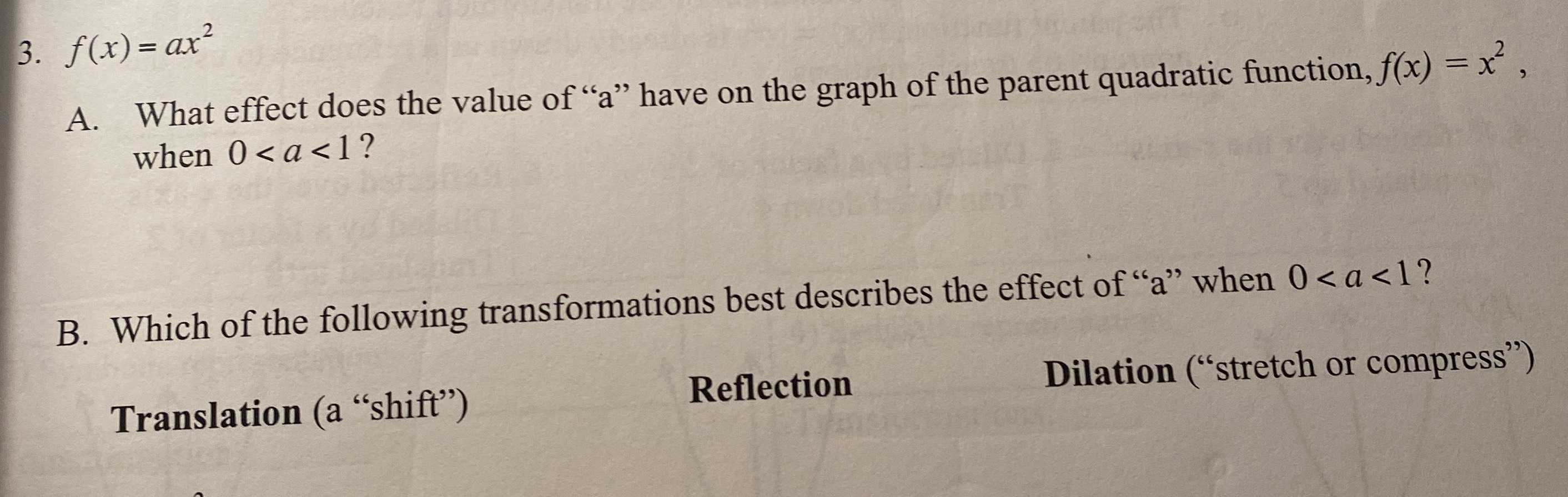### ¿Todavía tienes preguntas de matemáticas?

Pregunte a nuestros tutores expertos
Algebra
Pregunta3. $$f ( x ) = a x ^ { 2 }$$

A. What effect does the value of "a" have on the graph of the parent quadratic function, $$f ( x ) = x ^ { 2 }$$ , when $$0 < a < 1$$ ?

B. Which of the following transformations best describes the effect of "a" when $$0 < a < 1$$ ?

Translation (a "shift") Reflection  Deflection (stretch or compress")